# Creating a Bike Speedometer with GreenPAK and a Magnetic Sensor

Below we described all the consecutive steps of the project. However, you can access the IC design file of this project here. The design was created in free GUI-based GreenPAK™ Designer software.

This project explores designing a speedometer to measure and display the speed of a bicycle wheel using a magnetic sensor. The system should be able to interpret measurement in meters per second. To achieve this system, a sensor device is attached to the bicycle. The Dialog GreenPAK design then performs logical operations to update the output periodically, or simultaneously, to interpret measurement results using four binary digits. This application is designed to be precise up to 1 m/s.

The input of the system is a digital signal from a magnetic switch. The magnetic switch is used because, though both magnetic and optical sensors are known to be used in tachometers, magnetic switches are favorable for applications that have ambient light. This is critical for a solution that can be easily-affixed to a bicycle. In this application, every full rotation of the wheel represents 2 meters traveled (roughly the circumference of the wheel).

This project describes two designs, both of which have their pros and cons. The first design utilizes the frequency detector of the SLG46536 to determine the rotational speed of the bike. The second uses the typical RPM method of incrementing the number of pulses (full rotations) over a given period.

The key advantages to using GreenPAK are that it is smaller, lower cost, simpler, and easier to develop than most other solutions. Furthermore, the GreenPAK design for the bike speedometer can be modified into complicated projects, such as the internal speedometer for an electric bike or a moped. A microcontroller could be used instead, but the size and power consumption of the microcontroller can make it less appealing to a customer.

The system can be tested by inspecting the digital output signal. For this application, we use between four and six LEDs for visual representation of the data. Each LED represents a single bit of a binary sequence. The application has been tested by comparing the digital output of GreenPAK to various radial speeds of a wheel. To show this solution is appealing for the biking industry we can evaluate dimension, development capability, and other parameters of comparison.

# First Design: Frequency Detector

In terms of components, the SLG46536 is one of the more complex GreenPAK ICs with a large number of counter/delay/frequency detector blocks. The frequency detector of the counter blocks acts like a frequency filter and can be configured by set counter data. From the counter data, a signal is generated that determines if the incoming pulses occur more or less frequently than the given period. For this IC, there are 8-bit and 16-bit frequency detectors. The 16-bit frequency detectors generate more precise measurements. This application uses both types.

If the input frequency of the block is higher than the configured frequency, the frequency block will output a digital high. Two 16-bit CNT blocks, utilized as a frequency detector, are shown in Figure 1.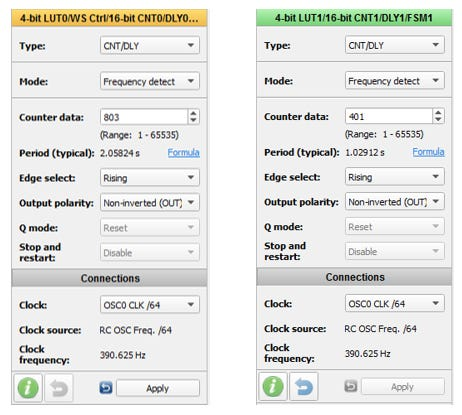Figure 1: Frequency Detector Configuration for Speed 1 m/s (Left Side) and 2 m/s (Right Side)

The application’s wheel circumference is 2.06 m. When the wheel of the bike rotates at least once in 2.060 s, the horizontal speed of the bike is more than 1 m/s. Because of that, the counter data is set to 803 with clock source OSC0 CLK/64. Using these calculations, the period of the block is 2.05824 s, as this is the closest configurable value to 2.060 s.

Similarly, to configure the second frequency detector to detect speeds of more than 2 m/s, the wheel of the bike should rotate at least once in 1.030 s. The counter data is therefore set to 401 with clock source OSC0 CLK/64, to make the period of the block 1.02912 s. This methodology will be implemented for the other speeds, that is, 3 m/s to 7 m/s, for a single IC in this application. The output of each frequency detector is given in Table 1.

To measure more than 7 m/s another IC can be added. The maximum speed is then 14 m/s. This speed is fast enough for a bike.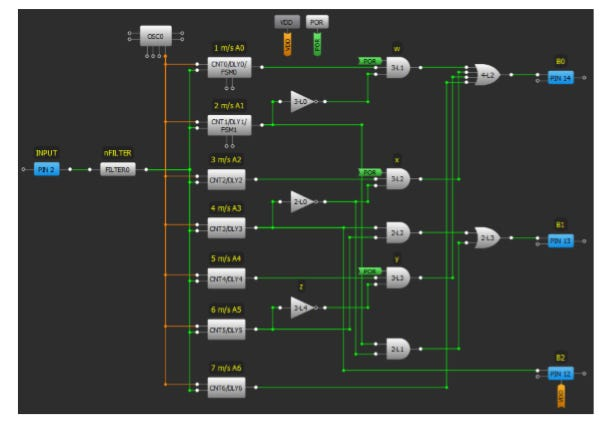Figure 2: GreenPAK Design for First Method with Measurement Range 0 to 7 m/s

Every 1 m/s increase in speed uses one counter block for detection. So, with only one IC, a maximum 7 m/s with a resolution of 1 m/s can be measured. The digital circuit on this design converts the frequency detector output bits (from Table 1) to a 3-bit binary sequence, which is output on B2:B0.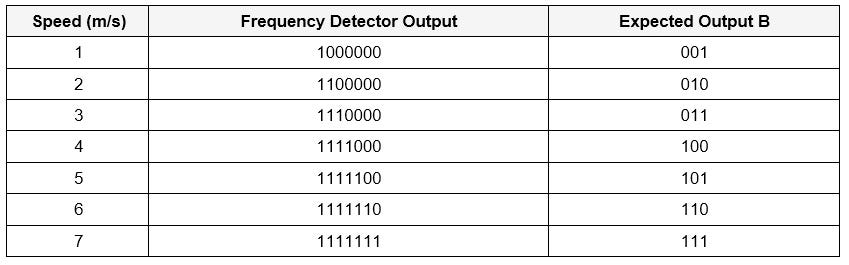Table 2: Input (Frequency Detector Output) and Output of Digital Circuit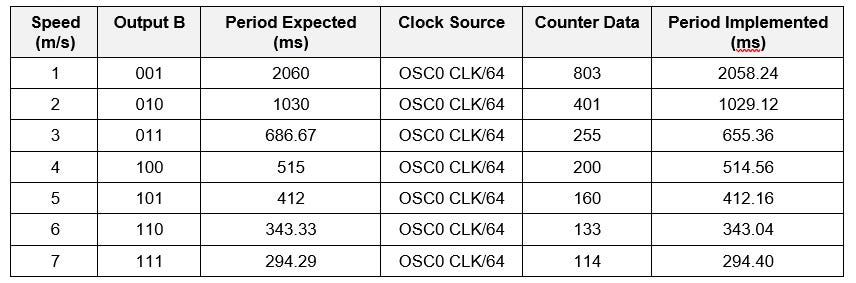Table 3: Configuration Table Using GreenPAK for 1 m/s to 7 m/s

## Extended Version of Speedometer with Speed Range up to 14 m/s

In the previous design, the maximum speed is only up to 7 m/s. Adding a second IC scales the maximum speed up to 14 m/s. Figure 3 shows a GreenPAK design and configuration that has been implemented with the SLG46536V.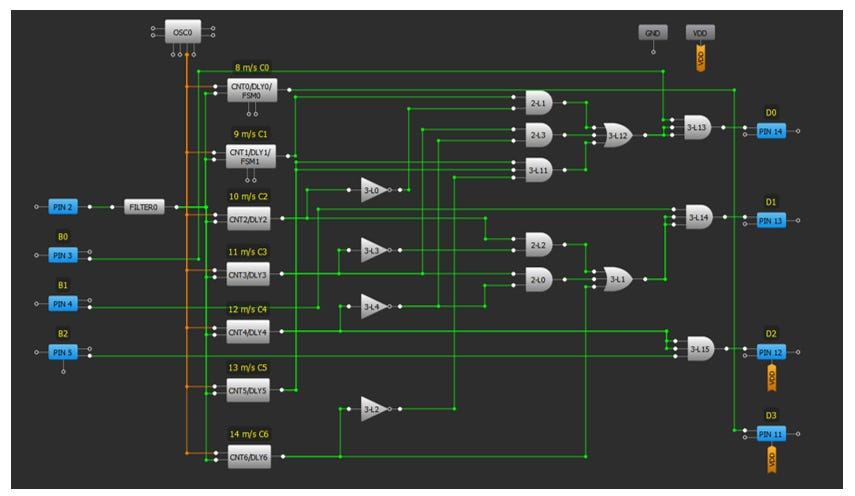Figure 3: GreenPAK Design to Extend the First Method with a Range of 8 m/s to 14 m/s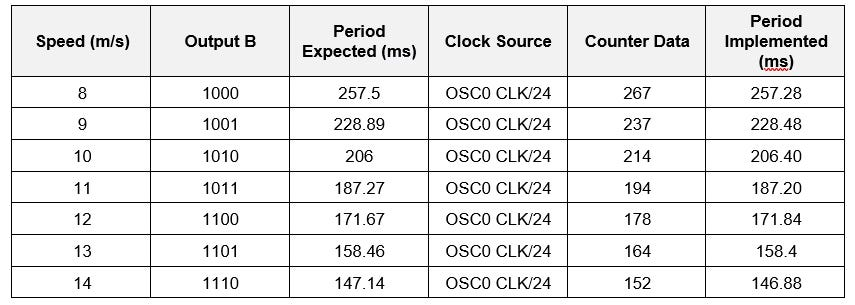Table 4: Configuration Table Using GreenPAK for 8 m/s to 14 m/s

Overall, the design for the extended IC is similar to the main IC. However, the output of the main IC is also an input of the extended IC. The overall system using two ICs for this application is shown in Figure 4.

# Second Design: RPM Counter

The second design is a very different method of reading speed than the first. This design uses a full‑adder digital circuit. Every rotation of the wheel increments the counter by one. The counter resets after a given period of time. This design uses 2.06 s as the period; this also becomes the refresh-rate of the measured result. So, if there is only one rotation in 2.06 s, the bike’s speed is only 1 m/s. If there are two rotations in 2.06 s, the bike’s speed is 2 m/s, and so on.

In this design, the maximum speed can be measured up to 63 m/s (6-bit output). It is possible to optimize this method based on requirements. For example, the measurement accuracy can be improved to 1 km/h; the output will update for every 2.06 x 3.6 = 7.42 s. Since this refresh rate would be unusable for many applications, another option would be to improve the accuracy up to 2 km/h and update the output every 3.71 s. So, the measurement result is multiplication of 2 km/h such as 2, 4, 6, 8, 10, and so on. The design can also be implemented with a 3 km/h accuracy and update the output every 2472 ms. The system diagram of this method is illustrated in Figure 6.

The simplest method to improve accuracy would be to have more than one magnet on the wheel; this would ensure multiple pulses per rotation and would give better resolution in RPM.

# Results

There are two possible designs for comparison.

In most circumstances, the second design is a better application, so the results focus on the second design using the RPM counter.

The back wheel of the bicycle is used to test the speedometer designs. The measurement results for each wheel speed are given below. There are five LEDs. The most significant bit (MSB) is interpreted by the LED on the left side.

Figure 10 shows the results for the first 4 bits (up to 7 m/s), where the design was able to output the binary value of the bike’s velocity.

An alternative solution to using GreenPAK would be to use a small microcontroller, such as an ATtiny85. However, using an MCU has its own disadvantages such as knowing how to program it, which programming environment to use, and increased cost and size, see Table 6.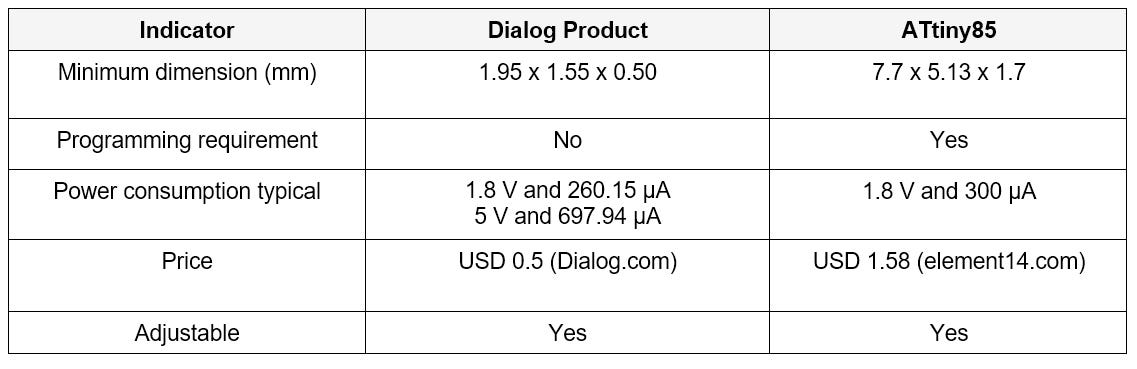Table 6: Comparison to Competing Solution with Reference to each Datasheet

# Conclusion

This project outlined how to create a simple bike speedometer with a Dialog GreenPAK SLG46536V. The rotational speed of the bicycle wheel was measured with a magnetic sensor. Two approaches to solving the problem were demonstrated — a frequency method and an RPM method. The RPM counter could also be used for other applications such as anemometers, tachometers, and so on.

--

--